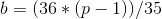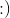# HELP MILO PROBLEM DOUBT

PROBLEM
my code
MY CODE
Why I am getting WA
I feel problem with some MOD operation
I want to calculate
36^x + ((36^x * (x) * (x + 1)) / 2) + ((36 * (36^x - 1)) / 35)

1 Like

your formula is wrong as i think

formula is wrong
I think u have wrongly applied the Gp formula if not then check the geometric series
Series is:-
36^(x){2+x(x+1)/2}+36^x-1+36^(x-2)+. . . . .+36
Ending at 36 and not 1.For n=0 this series will not give the result so print it seperately

You can solve it using matrix exponentiation also.

1 Like

CAN U TELL THE MATRIX ?

@aryanc403 please help me with this question … tell formula with some explainataionCan you please explain how to decide the matrix.
I saw few solution in which matrix is
{{36,36,36,1},{0,36,36,0},{0,0,36,0},{0,0,0,1}}

I cant get it.

The recursive function will be -
f(n+1) = 36f(n) + (n+1)(36^(n+1)) + 36, ( according to problem).
You can break this as-
f(n+1) = 36f(n) + n(36^(n+1)) + 36^(n+1) + 36 where f(n) is total number of estimated registrations on the given n’th day.
You can make a 4*4 matrix {36, 36 , 36 , 1 , 0 , 36 , 36 , 0 , 0 , 0 , 36 , 0 , 0,0,0,1}
and apply matrix exponentation.
You can check my solution.
extra materialFor those who want a (simple sa) formula- to cut the long story short:And if want explanation, ping me.

How to form transformation Matrix for such non linear recurrance relation ?
Here in this case its {{36,36,36,1},{0,36,36,0},{0,0,36,0},{0,0,0,1}}. How it is formed ?

1 Like

hey,
how you reached to these expressions,1 Like

explain plzzz1 Like

I’m in college. Will get back to you on reaching home.

I will try to explain theoretically first. See if you can pick it up.
You can obviously see the relation between nth term and (n-1)th term.
But you can’t use dp for that because n<=10^18
So, in that case it would be better, if you deduce the nth term expression.

Now, its a basic maths problem how to find nth term from a recurrence relation.
You can use summation method to find that.

Expressing the recurrence relation in form like nth term-(k*(n-1)th term) = f(n)
And then backtracking to 1th term and 0th term form.
And adding all them up, intermediate terms will cancel out.
And a nice handling of expressions will land you to the expressions I have posted.

my code
MY CODE
Why I am getting WA
I feel problem with some MOD operation
I have done the same
[/quote]